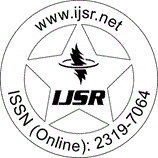International Journal of Science and Research (IJSR)
Call for Papers | Fully Refereed | Open Access | Double Blind Peer Reviewed

Case Studies | Engineering Science | Morocco | Volume 3 Issue 6, June 2014

# Using the Total Multiple Linear Regression and Artificial Neurons Network-Multi Layer Perceptron for Modelling the Normal System; at Variable Point of Functioning of a Continuous Distillation Column Methylcyclohexane

B. Boudebbouz | I. Manssouri | A. Mouchtachi | B. El Kihel

Abstract: This paper describes the modeling methods of normal functioning of a system of continuous distillation with variable working point of methylcyclohexane from a binary mixture (toluene / methylcyclohexane) using techniques of artificial Intelligence. Initially; the learning basis of the neural network was composed of 577 samples. In a second step; the weights and biases are determined by using the Levenberg Marquardt algorithm. Once the architecture; weights and biases of the neural network were established; it was necessary to know if the neural model is likely to be widespread. The validation of the neural architecture [7-14-1] is therefore to assess its ability to predict the temperature at the column head using the weights and biases calculated during learning; and apply them to another database tests basis composed of 384 data samples. The model of artificial neural network MLP-type [7-14-1] gave a factor of success for the testing and validation phase of 81.9 %. A comparative study was established between the neural model MLP type and classical statistical models; i. e. the total multiple linear regression.

Keywords: Modeling, Total multiple linear regression TMLR, Neural Networks MLP, continuous distillation column

Edition: Volume 3 Issue 6, June 2014,

Pages: 2829 - 2835